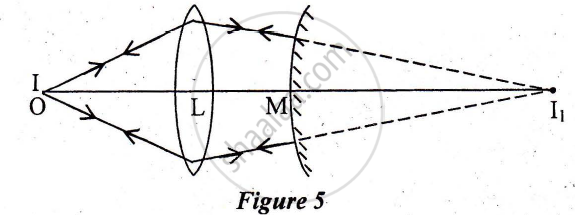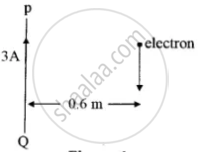# Question Bank Solutions for ISC (Arts) Class 12 - CISCE - Physics (Theory)

Subjects
Topics
Subjects
Popular subjects
Topics
Physics (Theory)
< prev  1 to 20 of 274  next >

Name a material which is used in making control rods in a nuclear reactor.

[0.08199999999999999] Nuclei
Chapter: [0.08199999999999999] Nuclei
Concept: Atomic Masses and Composition of Nucleus

Write one balanced equation to show Emission of beta^- (i.e. a negative beta particle)

[0.08199999999999999] Nuclei
Chapter: [0.08199999999999999] Nuclei
Concept: Atomic Masses and Composition of Nucleus

With the help of a suitable example and an equation, explain the term pair production.

[0.08199999999999999] Nuclei
Chapter: [0.08199999999999999] Nuclei
Concept: Atomic Masses and Composition of Nucleus

A converging lens of focal length 40 cm is kept in contact with a diverging lens of focal length 30 cm. Find the focal length of the combination .

[0.061] Ray Optics and Optical Instruments
Chapter: [0.061] Ray Optics and Optical Instruments
Concept: Reflection of Light by Spherical Mirrors

How can the spherical aberration produced by a lens be minimized?

[0.061] Ray Optics and Optical Instruments
Chapter: [0.061] Ray Optics and Optical Instruments
Concept: Reflection of Light by Spherical Mirrors

Two thin lenses having optical powers of -10D and+ 6D are placed in contact with each other. The focal length of the combination is:

[0.061] Ray Optics and Optical Instruments
Chapter: [0.061] Ray Optics and Optical Instruments
Concept: Reflection of Light by Spherical Mirrors

State how the focal length of a glass lens (Refractive Index 1.5) changes when it is completely immersed in:

(i) Water (Refractive Index 1.33)
(ii) A liquid (Refractive Index 1.65)

[0.061] Ray Optics and Optical Instruments
Chapter: [0.061] Ray Optics and Optical Instruments
Concept: Reflection of Light by Spherical Mirrors

A thin converging lens of focal length 12 cm is kept in contact with a thin diverging lens of focal length 18 cm. Calculate the effective/equivalent focal length of the combination.

[0.061] Ray Optics and Optical Instruments
Chapter: [0.061] Ray Optics and Optical Instruments
Concept: Reflection of Light by Spherical Mirrors

A circular coil carrying a current I has radius R and number of turns N. If all the three, i.e. the current
I, radius R and number of turns N are doubled, then, the magnetic field at its centre becomes:

(a) Double

(b) Half

(c) Four times

(d) One fourth

[0.031] Moving Charges and Magnetism
Chapter: [0.031] Moving Charges and Magnetism
Concept: Magnetic Force

An object is kept on the principal axis of a concave mirror of focal length 10 cm. at a distance of 15
cm from its pole. The image formed by the mirror is:

(a) Virtual and magnified

(b) Virtual and diminished

(c) Real and magnified

(d) Real and diminished

[0.061] Ray Optics and Optical Instruments
Chapter: [0.061] Ray Optics and Optical Instruments
Concept: Ray Optics - Mirror Formula

An emf of 2V is induced in a coil when the current in it is changed from 0A to 10A in 0·40 sec. Find the coefficient of self-inductance of the coil.

[0.040999999999999995] Electromagnetic Induction
Chapter: [0.040999999999999995] Electromagnetic Induction
Concept: Electromagnetic Induction

Explain the term hysteresis

[0.031] Moving Charges and Magnetism
Chapter: [0.031] Moving Charges and Magnetism
Concept: Magnetic Force

Obtain an expression for magnetic flux density B at the centre of a circular coil of radius R, having N turns and carrying a current I

[0.031] Moving Charges and Magnetism
Chapter: [0.031] Moving Charges and Magnetism
Concept: Magnetic Force

A point object O is placed at a distance of 15cm from a convex lens L of focal length 1 Ocm as shown in Figure 5 below. On the other side of the lens, a convex mirror M is placed such that its distance from the lens is equal to the focal length of the lens. The final image formed by this combination is observed to coincide with the object O. Find the focal length of the convex mirror[0.061] Ray Optics and Optical Instruments
Chapter: [0.061] Ray Optics and Optical Instruments
Concept: Ray Optics - Mirror Formula

A radioactive substance decays to 1/16th of its initial mass in 40 days. The half-life of the substance, in days, is:

[0.08199999999999999] Nuclei
Chapter: [0.08199999999999999] Nuclei

With what type of source of light are cylindrical wave fronts associated?

[0.062] Wave Optics
Chapter: [0.062] Wave Optics
Concept: Huygens Principle

The total energy of an electron in the ground state of the hydrogen atom is -13·6 eV. Its total energy, when a hydrogen atom is in the first excited state, is:

[0.081] Atoms
Chapter: [0.081] Atoms
Concept: Alpha-particle Scattering and Rutherford’s Nuclear Model of Atom

Write the expression for the Lorentz force F in vector form.

[0.031] Moving Charges and Magnetism
Chapter: [0.031] Moving Charges and Magnetism
Concept: Magnetic Force

A coil has a self-inductance of 0·05 Henry. Find the magnitude of the emf induced in it when the current flowing through it is changing at the rate of 100 As-1.

[0.040999999999999995] Electromagnetic Induction
Chapter: [0.040999999999999995] Electromagnetic Induction
Concept: Electromagnetic Induction

PQ is a long straight conductor carrying a current of 3A as shown in Figure below. An electron moves with a velocity of 2 x 107 ms-1 parallel to it. Find the force acting on the electron.[0.031] Moving Charges and Magnetism
Chapter: [0.031] Moving Charges and Magnetism
Concept: Magnetic Force
< prev  1 to 20 of 274  next >